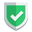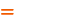我们相信：世界是美好的，你是我也是。平行空间的世界里面，不同版本的生活也在继续...

## 基本情况

``````_list = [ expression for_loop_expression if condition ]
_set  = { expression for_loop_expression if condition }
_tuple= tuple(( expression for_loop_expression if condition ))
_dict = { key_expr:value_expr for_loop_expression if condition }``````
• `expression`函数或者表达式，用于加工集合中的某个成员。
• `for_loop_expression`，用于产生集合成员的初始值。
• `if condition`，用于数据筛选。如果为真，则从`for`流程转到`expression`流程。

## 测试代码一

``````_test = range(0, 10)
def fn(i):
return i*i
_list = [i*i for i in _test if i % 2 == 0]
_set = {fn(i) for i in _test }
_tuple = tuple((i*i for i in _test if i % 2 ==0))
_map = map(fn,_test) ``````
• 其中的列表`list`和集合`set`的推导式，中规中矩。
• 对于`tuple`元组，使用`()`表述得到的是个生成式。然后使用了强转。
• 对于`map`类型，这个可能不叫做推导式了，但是表现形式上和推导式非常类似。

``````_list = []
for i in _test:
if i % 2 == 0:
_list.append(i)
print(_list)``````

## 测试代码二

``````_info = {'sunan': {'grade': "A",},'sunan2': {'grade': "B",}}
_dict = {o:d for o,d in _info.items() if d['grade'] == "A"}
print(_dict, type(_dict))``````

## 综述如果本文对您有帮助，或者节约了您的时间，欢迎打赏瓶饮料，建立下友谊关系。本博客不欢迎：各种镜像采集行为。请尊重原创文章内容，转载请保留作者链接。【福利】 腾讯云最新爆款活动！1核2G云服务器首年50元！【源码】本文代码片段及相关软件，请点此获取更多信息【绝密】秘籍文章入口，仅传授于有缘之人python